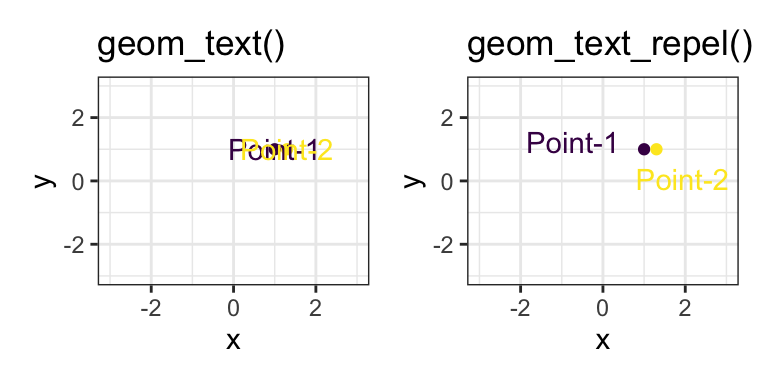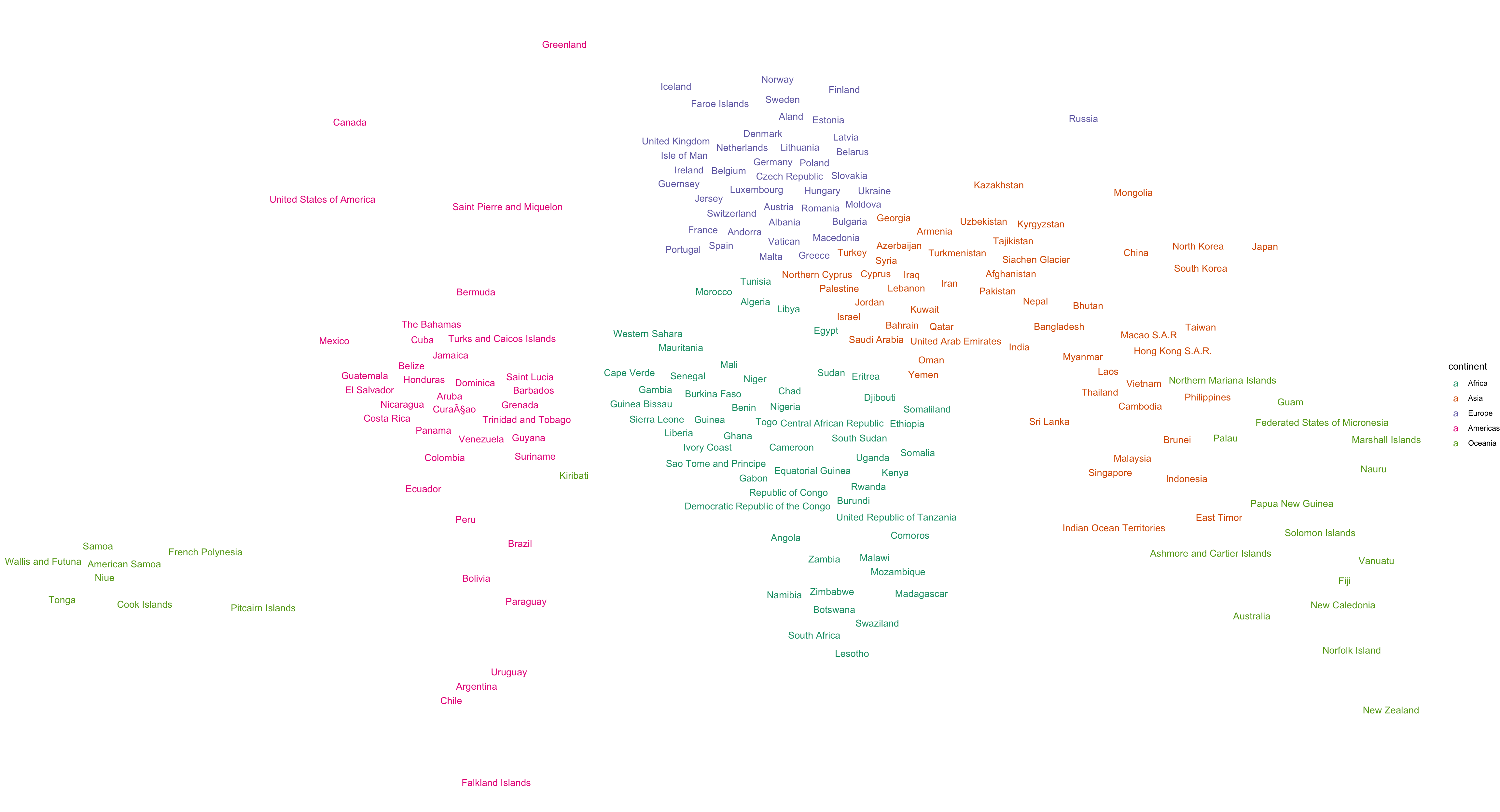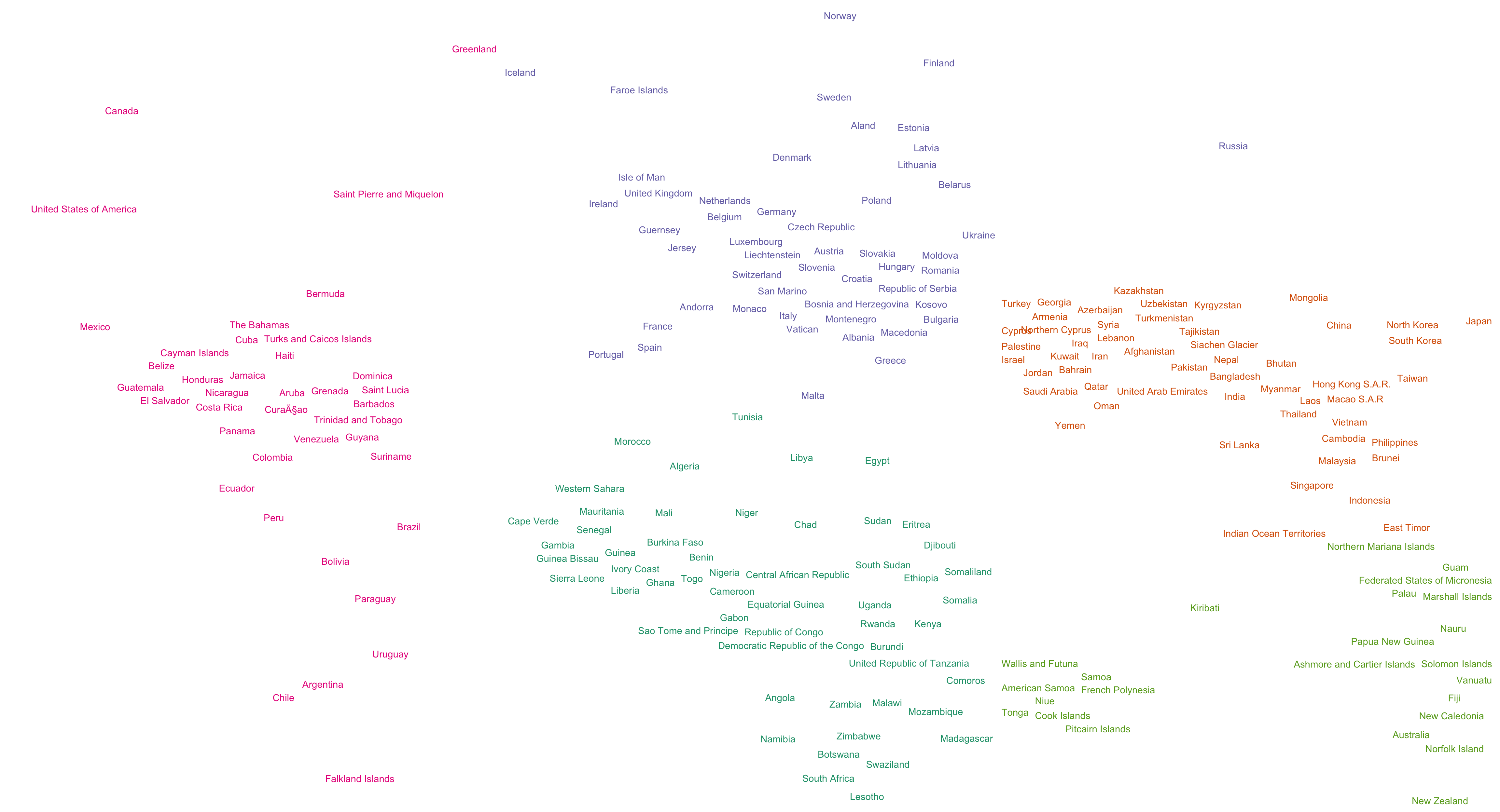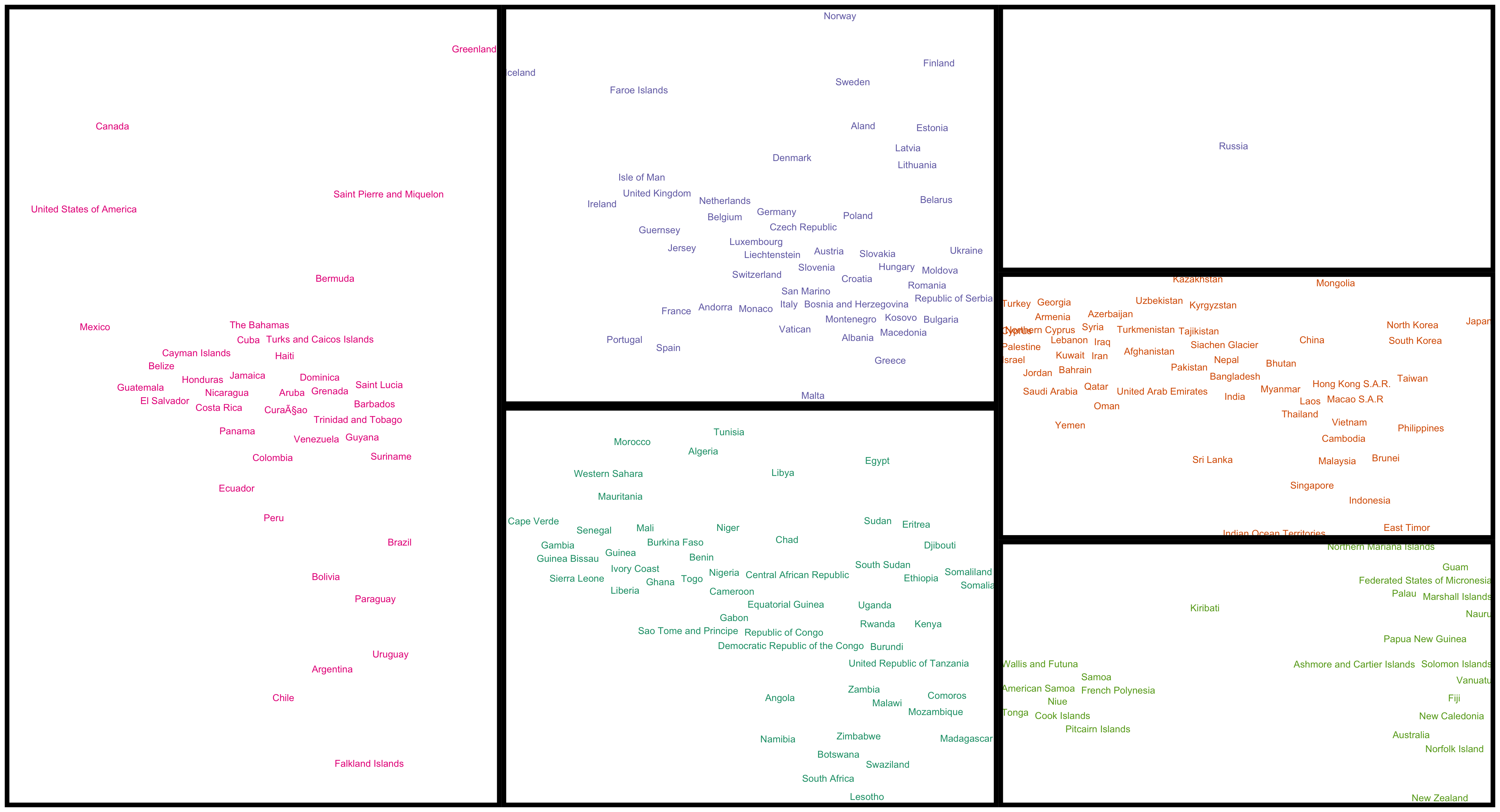This post demonstrates the use of two very cool R packages - `ggrepel` and `patchwork`.

`ggrepel` deals with overlapping text labels (Code#1 at the bottom of this post):`patchwork` is a very convenient new package for combining multiple different plots together (i.e. what we usually to use `grid` and `gridExtra` for).

To really demonstrate the power of them, let’s make a global map of country names using `ggrepel`:

``````library(tidyverse)
library(ggrepel)
library(patchwork)

# data from https://worldmap.harvard.edu/data/geonode:country_centroids_az8

centroidsdata = orig_data %>%
select(country = admin, continent, lat = Latitude, lon = Longitude) %>%
filter(continent != "Seven seas (open ocean)" & continent != "Antarctica") %>%
mutate(continent  = fct_collapse(continent, "Americas" = c("North America", "South America")))

``````## # A tibble: 6 × 4
##   country     continent   lat   lon
##   <chr>       <fct>     <dbl> <dbl>
## 1 Aruba       Americas   12.5 -70.0
## 2 Afghanistan Asia       33.8  66.0
## 3 Angola      Africa    -12.3  17.5
## 4 Anguilla    Americas   18.2 -63.1
## 5 Albania     Europe     41.1  20.0
## 6 Aland       Europe     60.2  20.0``````
``````plot1 = centroidsdata %>%
ggplot(aes(x = lon, y = lat, label = country, colour = continent)) +
geom_text_repel(segment.alpha = 0)   +
theme_void() +
scale_color_brewer(palette = "Dark2")

plot1``````
``````## Warning: ggrepel: 24 unlabeled data points (too many
## overlaps). Consider increasing max.overlaps``````Now this is very good already with hardly any overlapping labels and the world is pretty recognisable. And really, you can make this plot with just 2 lines of code:

``````ggplot(centroidsdata, aes(x = lon, y = lat, label = country)) +
geom_text_repel(segment.alpha = 0)``````

So what these two lines make is already very amazing.

But I feel like Europe is a little bit misshapen and that the Caribbean and Africa are too close together. So I divided the world into regions (in this case same as continents except Russia is it’s own region - it’s just so big). Then wrote two functions that asked `ggrepel` to plot each region separately and use `patchwork` to patch each region together:

``````centroidsdata = centroidsdata %>%
mutate(region = continent %>% fct_expand("Russia")) %>%
mutate(region = replace(region, country == "Russia", "Russia"))

mapbounds = centroidsdata %>%
group_by(region) %>%
summarise(xmin = min(lon), xmax = max(lon), ymin = min(lat), ymax = max(lat))

create_labelmap = function(mydata, mycontinent, myforce = 1, mycolour = "black"){

mymapbounds = mapbounds %>%
filter(region == mycontinent)

mydata %>%
filter(region == mycontinent) %>%
ggplot(aes(x = lon, y = lat, label = country)) +
geom_text_repel(segment.alpha = 0, force = myforce, colour = mycolour)   +
theme_void() +
theme(legend.position = "none") +
scale_y_continuous(limits = c(mymapbounds\$ymin, mymapbounds\$ymax)) +
scale_x_continuous(limits = c(mymapbounds\$xmin, mymapbounds\$xmax))

}

mycolours = RColorBrewer::brewer.pal(5,"Dark2")

make_world = function(mydata){
mydata  = centroidsdata
afr = create_labelmap(mydata, "Africa",   mycolour = mycolours)
ame = create_labelmap(mydata, "Americas", mycolour = mycolours)
asi = create_labelmap(mydata, "Asia",     mycolour = mycolours)
eur = create_labelmap(mydata, "Europe",   mycolour = mycolours)
rus = create_labelmap(mydata, "Russia",   mycolour = mycolours)
oce = create_labelmap(mydata, "Oceania",  mycolour = mycolours)

(ame + (eur / afr) + (rus / asi / oce)) + plot_layout(ncol = 3)
}

plot2 = make_world(centroidsdata)

plot2``````
``````## Warning: ggrepel: 12 unlabeled data points (too many
## overlaps). Consider increasing max.overlaps``````This gives continents a much better shape, but it does severaly misplace Polynesia. See if you can find where, e.g., Tonga is and where it should be.

To see what I did with patchwork there, let’s add black borders to each region (Code#2):

``````## Warning: ggrepel: 12 unlabeled data points (too many
## overlaps). Consider increasing max.overlaps``````Code#1:

``````#devtools::install_github("slowkow/ggrepel")
#devtools::install_github("thomasp85/patchwork")

library(tidyverse)
library(ggrepel)
library(patchwork)

mydata  = data_frame(x = c(1, 1.3), y = c(1, 1), mylabel = c("Point-1", "Point-2"))

p = mydata %>%
ggplot(aes(x, y, label = mylabel, colour = mylabel)) +
geom_point() +
coord_cartesian(xlim = c(-3, 3), ylim = c(-3, 3)) +
theme_bw() +
theme(legend.position = "none") +
scale_colour_viridis_d()

plot1 = p + geom_text() + ggtitle("geom_text()")

plot2 = p+ geom_text_repel() + ggtitle("geom_text_repel()")

plot1 + plot2``````

Code#2:

``````create_labelmap = function(mydata, mycontinent, myforce = 1, mycolour = "black"){

mymapbounds = mapbounds %>%
filter(region == mycontinent)

mydata %>%
filter(region == mycontinent) %>%
ggplot(aes(x = lon, y = lat, label = country)) +
geom_text_repel(segment.alpha = 0, force = myforce, colour = mycolour)   +
theme_void() +
theme(legend.position = "none") +
scale_y_continuous(limits = c(mymapbounds\$ymin, mymapbounds\$ymax)) +
scale_x_continuous(limits = c(mymapbounds\$xmin, mymapbounds\$xmax)) +
theme(panel.border = element_rect(colour = "black", fill=NA, size=5))

}

plot3 = make_world(centroidsdata)

plot3``````